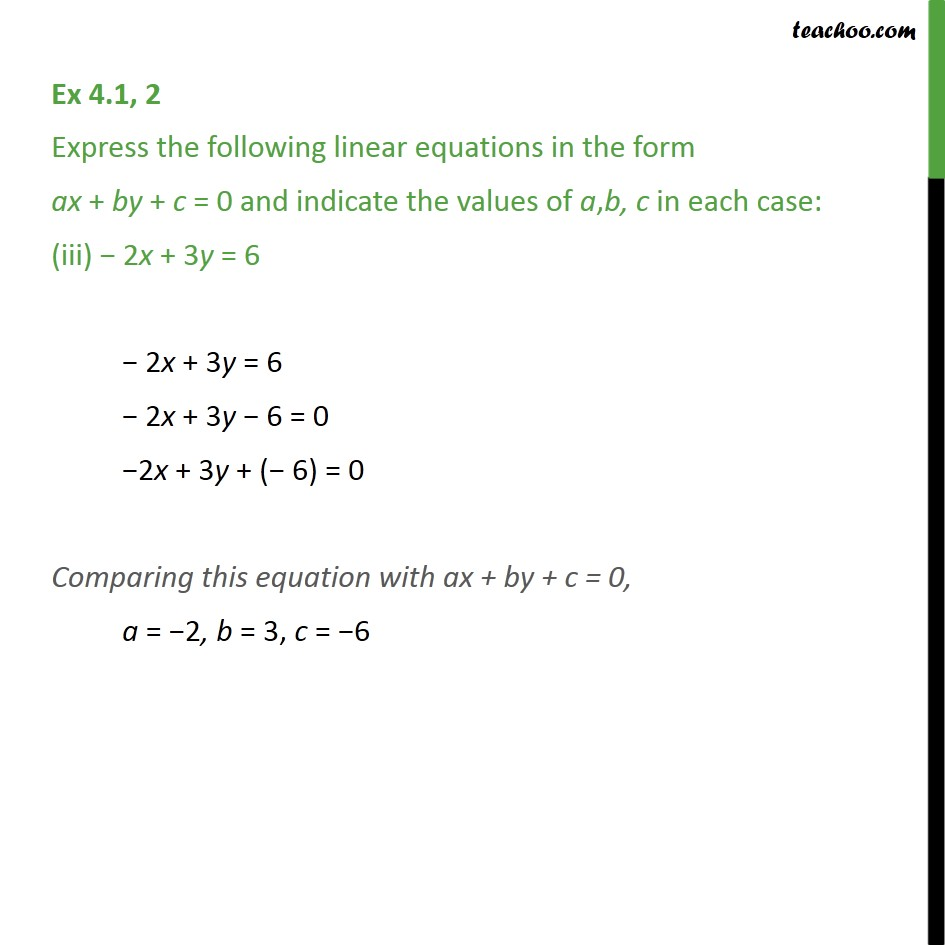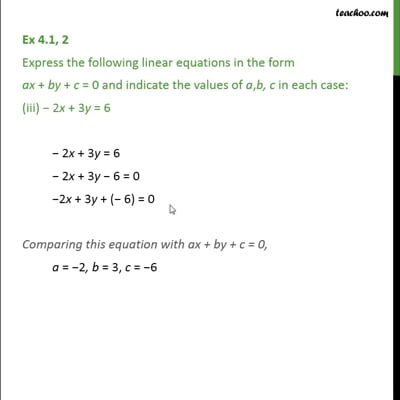Ex 4.1

Chapter 4 Class 9 Linear Equations in Two Variables
Serial order wiseThis video is only available for Teachoo black users

Introducing your new favourite teacher - Teachoo Black, at only ₹83 per month

### Transcript

Ex 4.1, 2 Express the following linear equations in the form ax + by + c = 0 and indicate the values of a,b, c in each case: (iii) − 2x + 3y = 6 − 2x + 3y = 6 − 2x + 3y − 6 = 0 −2x + 3y + (− 6) = 0 Comparing this equation with ax + by + c = 0, a = −2, b = 3, c = −6